• +91 9971497814
• info@interviewmaterial.com

# Chapter 3 Trigonometric Functions Ex-3.4 Interview Questions Answers

### Related Subjects

Question 1 : Find the principal and general solutions of the equation  tan x = √3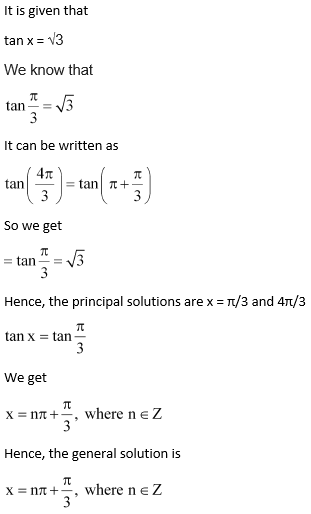Question 2 : Find the principal and general solutions of the equation sec x = 2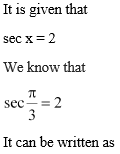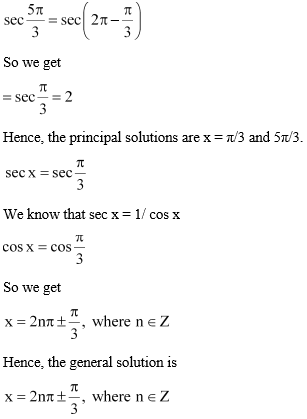Question 3 : Find the principal and general solutions of the equation cot x = – √3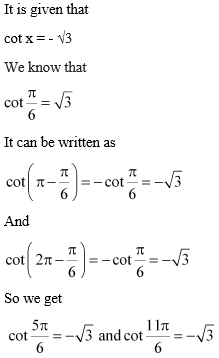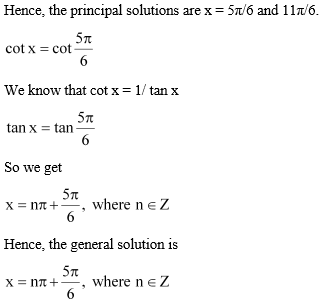Question 4 : Find the principal and general solutions of the equation cosec x = – 2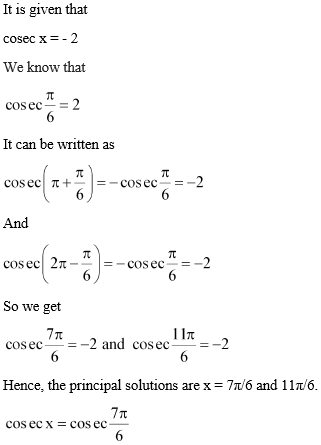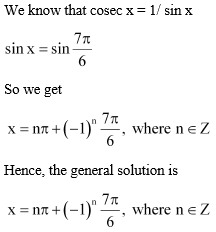Question 5 : Find the general solution of the equation cos 4x = cos 2x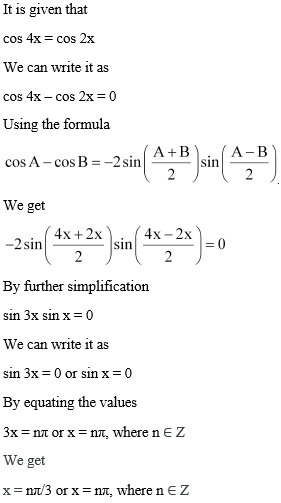Question 6 : Find the general solution of the equation cos 3x + cos x – cos 2x = 0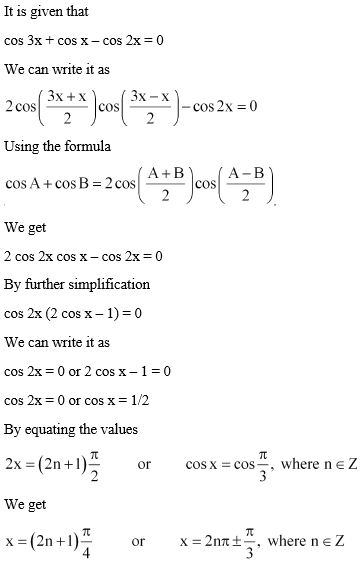Question 7 : Find the general solution of the equation   sin 2x + cos x = 0

It is given that
sin 2x + cos x = 0
We can write it as
2 sin x cos x + cos x = 0
cos x (2 sin x + 1) = 0
cos x = 0 or 2 sin x + 1 = 0
Let cos x = 0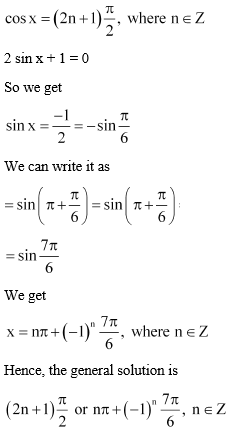Question 8 : Find the general solution of the equation   sec2 2x = 1 – tan 2x

It is given that

sec2 2x= 1 – tan 2x

We can write it as

1 + tan2 2x= 1 – tan 2x

tan2 2x+ tan 2x = 0

Taking common terms

tan 2x (tan 2x + 1) =0

Here

tan 2x = 0 or tan 2x +1 = 0

If tan 2x = 0

tan 2x = tan 0

We get

2x = nπ + 0, where n Z

x = nπ/2, where n Z

tan 2x + 1 = 0

We can write it as

tan 2x = – 1

So we get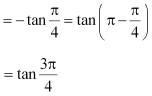Here

2x = nπ + 3π/4, wheren Z

x = nπ/2 + 3π/8, wheren Z

Hence, the generalsolution is nπ/2 or nπ/2 + 3π/8, n Z.

Question 9 : Find the general solution of the equation  sin x + sin 3x + sin 5x = 0

It is given that
sin x + sin 3x + sin 5x = 0
We can write it as
(sin x + sin 5x) + sin 3x = 0
Using the formula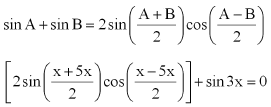By further calculation

2 sin 3x cos (-2x) +sin 3x = 0

It can be written as

2 sin 3x cos 2x + sin3x = 0

By taking out thecommon terms

sin 3x (2 cos 2x + 1)= 0

Here

sin 3x = 0 or 2 cos 2x+ 1 = 0

If sin 3x = 0

3x = nπ, where n Z

We get

x = nπ/3, where n Z

If 2 cos 2x + 1 = 0

cos 2x = – 1/2

By furthersimplification

= – cos π/3

= cos (π – π/3)

So we get

cos 2x = cos 2π/3

Here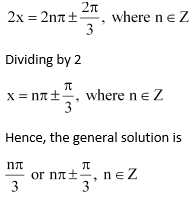Todays Deals### Chapter 3 Trigonometric Functions Ex-3.4 Contributorskrishan

Name:
Email:

# Latest News# 9000 interview questions in different categories